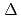Annular cores were formed in startup-core-physics tests of the High Temperature Engineering Test Reactor (HTTR) to obtain experimental data for verification of calculation codes. The first criticality, control rod positions at critical conditions, neutron flux distribution, excess reactivity etc. were measured as representative data. These data were evaluated with Monte Carlo code MVP that can consider the heterogeneity of coated fuel particles (CFP) distributed randomly in fuel compacts directly. It was made clear that the heterogeneity effect of CFP on reactivity for annular cores is smaller than that for fully-loaded cores. Measured and calculated effective multiplication factors (k) were agreed with differences less than 1%k. Measured neutron flux distributions agreed with calculated results. The revising method was applied for evaluation of excess reactivity to exclude negative shadowing effect of control rods. The revised and calculated excess reactivity agreed with differences less than 1%k/k.